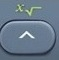# Cube Root

Cube Root = ³Number³ = ³Number × Itself × Itself

Basics
Cube Root = ³Number³ = ³Number × Itself × Itself

Cube Root of 27 = ³27 = ³ = ³3 × 3 × 3 = 3
Cube Root of 1728 =  ³1728 = ³12³ = ³12 × 12 × 12 = 12
Cube Root of 4657.463 = ³4657.463 = ³16.7³ = ³16.7 × 16.7 × 16.7 = 16.7

The root sign √   is also known as the radical sign.is the cube root command.activatesabove.

Formulas (NOT provided by Mathematics Formula Sheet)
Cube Root = ³Number³ = ³Number × Itself × Itself

Question
What is ³64 ?

4

Calculator Click What You See Commentblinker clears screen64 ³64 Cube Root4 Answer
###### Cube Root

Question
What is ³1906.624 ?

12.4

Calculator Click What You See Commentblinker clears screen1906.624 ³1906.624 Cube Root12.4 Answer
###### Cube Root

Practice – Questions
1.  What is the cube root of 729?

2.  What is the cube root of 15625?

3.  What is the cube root of 1,000,000,000?

4.  What is the cube root of 5088.448?

5.  What is the cube root of 2352.637?